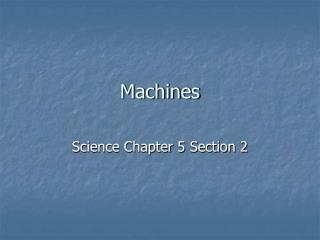DownloadDownload PresentationMachines

# Machines

Télécharger la présentation## Machines

- - - - - - - - - - - - - - - - - - - - - - - - - - - E N D - - - - - - - - - - - - - - - - - - - - - - - - - - -
##### Presentation Transcript

1. Machines Science Chapter 5 Section 2

2. Machine • Definition: A device that makes work easier • Does NOT decrease work! • Makes work easier by: • Changing direction of force needed to do work • Changing distance force is applied (also changes amount of force)

3. Change direction of Force

4. Change distance force is applied

5. Machine • Two forces are involved in using machine: • Effort force (FE): the force applied to the machine • Resistance force (FR): the force the machine uses on the object we want to move • FR is also sometimes called load force

6. Machine • Two kinds of work calculated when using the machine: • Input Work: Work done by you to use the machine (Win) • Output Work: Work done by the machine to move the object (Wout)

7. Remember! • Work is done by transferring energy! • We cannot create or destroy energy! • Some energy becomes non-useful any time work is done! • Therefore, Wout is always less than Win!

8. Mechanical Advantage • MA: the amount a machine helps you do the work • Calculated in two ways: • Ideal Mechanical Advantage • Actual Mechanical Advantage

9. Ideal Mechanical Advantage • IMA = deffort / dresistance • This is theoretical MA • Does not take “loss” of energy into account

10. Actual Mechanical Advantage • AMA = Fresistance / Feffort • “Real World” mechanical advantage • Takes friction and “loss” of energy into account

11. Example • Grok uses a ramp to push a 1500 N rock up to his cave from the valley below. The vertical distance from the valley floor to the cave is 50 m. The ramp is 150 m long, and Grok must push with a force of 500 N to move the rock up the ramp. What is the IMA of the ramp?

12. Grok’s Rock Example Continued • What we know: • Feffort=500 N • Fresistance=1500 N • deffort=150 m • dresistance=50 m • Equation • IMA = deffort / dresistance

13. Grok’s Rock Example • Substitute numbers in: • IMA = 150m/50m • Solve: • IMA = 3 • Check for units! • Whoops, no units for MA!

14. Efficiency! • Efficiency is another measure of how well the machine works • Efficiency compares the work you get out to the work you put in • Efficiency = Wout/Win x 100 (given as percent) • Efficiency is ALWAYS less than 100%!